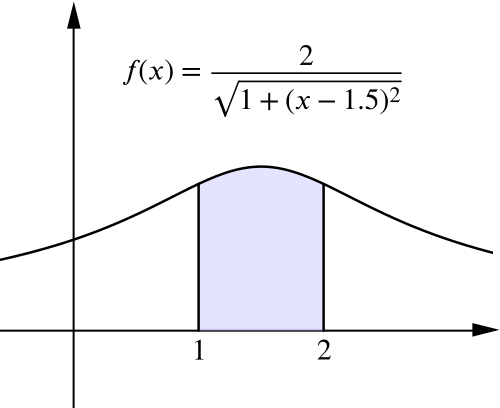Food for thought

## Things you might have noticed

What can you say about the area under each function on the two cards below, between $x=1$ and $x=2$?

Which is greater?

 (a) $f(x)=\left(4-x^2\right)^{\frac{1}{2}}$ (b)#### Card (a)

The given function represents a semi-circle of radius $2$, centred on the origin. The area we are concerned with is shaded in purple.

#### Cards (a) & (b)

It makes sense to begin our comparison by plotting the two functions on the same axes. Based on our subsequent observations we can think about accuracy and perhaps consider alternative approaches as a comparison.

• How can you convince someone that the maximum value of each function is the same?

• What can you say about the area under each function on the two cards between $x=1$ and $x=2$?